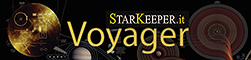# Automatic focusing from DragScript at the start of a session

Hi all,

I have a telescope, which focus heavily depends on the scope temperature. Sometimes the temperature difference between sessions is so big, that the scope is seriously out of focus. In this case RoboStar can not point to a focus star, because plate solving does not work. So automatic focusing fails.

I have a mobile setup and I usually had to pre-focus manually, before running my DragScript.

Starting with Voyager version 2.2.9d it is possible to automatically pre-focus from a DragScript. Thanks to Leo for implementing a “Multiply Decimal Number” command.

The idea is to get focusing results from Voyager log files. I.e. search for the following lines:

`````` Position = 14646  Mean HFD =  8.17  Temperature = 8.81
``````

And then find how the focuser position depends on the focuser temperature. In other words, compute coefficients A and B in the following equation:
FocuserPosition = A * FocuserTemperature + B

Then the following DragScript block will perform an initial focusing (-323.6 and 16570 are values for my system):

``````30  - 		Block: Initial focus
31  - 			Remark: ==========================================================
32  - 			Remark: --- Initial autofocus
33  - 			Goto ALT/AZ: ALT 80 00 00 - AZ 160 00 00 - FC: False
34  - 			Start Tracking
35  - 			Block: Pre-focus
36  - 				Remark: ----------------------------------------------------
37  - 				Remark: Set an approximate focuser position in order to make the plate-solving working for RoboStar
38  - 				Remark: FocuserPosition = -323.6 * FocuserTemperature + 16570
39  - 				Decimal Number: FocuserPosition - Init Value = 0
40  - 				Update Decimal from Focuser Temperature: FocuserPosition
41  - 				Multiply Decimal Number: FocuserPosition - Multiply by Value -323.6
42  - 				Update Decimal Number: FocuserPosition - Offset by 16570
43  - 				RoboFire Focuser Move From Variable: Label=FocuserPosition - Abs
44  - 			Precise Pointing RoboStar Star: Bayer Matrix
45  - 				IF OK
46  - 					AutoFocus with RoboStar: Bayer Matrix - AutoFocus OnPlace
47  - 						IF OK
48  - 							Goto Block: Start imaging
49  - 			Wait Time: 00:01:00 [hh:mm:ss] Interval
50  - 			Repeat Block Until Astronomical Night End: Data from connected Setup - Offset (Before) 00:30:00 [hh:mm:ss]
51  - 			Goto Block: Terminate Session
52  - 		Block: Start imaging
``````

The block ‘Pre-focus’ sets the initial focuser position.

I’ve prepared a python script, which parses Voyager logs and computes coefficients A and B. It requires Python 3.8, as well as matplotlib and scipy packages.

``````# Find the focusing results in the Voyager logs:
# Position = 14646  Mean HFD =  8.17  Temperature = 8.81
#
# Print A and B from the equation:
# FocuserPosition = A * FocuserTemperature + B

from os import listdir
from os.path import isdir, isfile, join
from pathlib import Path
import numpy as np
import matplotlib.pyplot as plt
from scipy.optimize import curve_fit
import re

# Fitting function for curve_fit
def func(x, a, b):
return a*x + b

# Extract floating point numbers from a string
def findNumbers(value):
return [float(v) for v in re.findall(r'[+|-]?\d*\.\d+|\d+', value)]

# Voyager log directory default location
LogDir = join(str(Path.home()), 'Documents/Voyager/Log')
if not isdir(LogDir):
print('No log')
quit()

# Read the list of log files: 2020_04_08_Voyager.log
Files = [f for f in listdir(LogDir) if isfile(join(LogDir, f))]
LogFiles = []
for name in Files:
if '_Voyager.log' in name:
LogFiles.append(name)

if len(LogFiles) <= 0:
print('No log')
quit()

# Read all log files and store focusing results in arrays
xdata = [] # Temperature
ydata = [] # Focuser position

for LogFile in LogFiles:
# Read the log lines
lines = 0
with open(join(LogDir, LogFile), 'r') as f:
lines = f.read().splitlines()
if len(lines) <= 0:
continue

# Search for 'Position = 14646  Mean HFD =  8.17  Temperature = 8.81'
for i in range(0, len(lines) - 1):
if 'Position =' in lines[i] and 'Mean HFD =' in lines[i] and 'Temperature =' in lines[i]:
parts = lines[i].partition('Position =')
if parts != '':
valueFloats = findNumbers(parts)
xdata.append(valueFloats) # Temperature
ydata.append(valueFloats) # Position
#print(str(valueFloats) + ',' + str(valueFloats) + ',' + str(valueFloats))

# Curve fitting
popt, pcov = curve_fit(func, xdata, ydata)
print(str(popt) + ", " + str(popt))

# Plot the data
plt.plot(xdata, ydata, 'bo')
plt.plot(xdata, func(np.asarray(xdata), *popt), 'r-')
plt.xlabel('Focuser temperature, C')
plt.ylabel('Focuser position')
plt.legend(['Focuser position', 'Position = A * T + B, A=%5.1f, B=%5.1f' % tuple(popt)], loc='lower left')
plt.show()
``````

Hopefully this will be useful for someone.

Thanks,
Vitali

7 Likes

Great job Vitali, this is a great post.
Thanks for sharing with us your script and code.

Just for my internal info, have you tried with LocalField focus that not need pointing ?

All the best
Leo

Thanks Leo. Yes, I’ve tried to use LocalField initially. However it did not work reliable, so I’ve switched to the RoboStar method.
One of the issues with LocalField was that in some part of the sky it could not get enough stars. Because my telescope has a narrow field of view (28x19 arcmin).

Best regards,
Vitali

1 Like